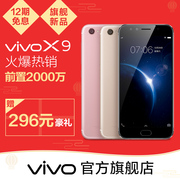# 【正品】vivo手机原装USB充电器/充电头2000mA 适用XPlay3S/Y35A

¥68.00
38人评价

1. 本平台不售卖商品，请点击直达链接进入vivo官方旗舰店进行购买
• 描述：4.8

• 物流：4.8

• 服务：4.8

• {"list": [{"k":"规格参数","v":[{"k":"拍照功能","v":[{"k":"后置摄像头","v":"1600万"},{"k":"摄像头类型","v":"三摄像头"},{"k":"视频显示格式","v":"1080P(全高清D5)"}]},{"k":"显示","v":[{"k":"屏幕尺寸","v":"5.5英寸"},{"k":"触摸屏类型","v":"电容式"},{"k":"分辨率","v":"1920*1080"}]},{"k":"网络","v":[{"k":"网络类型","v":"4G全网通"},{"k":"网络模式","v":"双卡多模"}]},{"k":"机身详情","v":[{"k":"款式","v":"直板"},{"k":"键盘类型","v":"虚拟触屏键盘"},{"k":"厚度","v":"9mm以下"}]},{"k":"存储","v":[{"k":"运行内存RAM","v":"4GB"},{"k":"存储容量","v":"128GB64GB"}]},{"k":"基本参数","v":[{"k":"CPU品牌","v":"高通"},{"k":"CPU型号","v":"MSM8953"},{"k":"产品名称","v":"X9"},{"k":"品牌","v":"vivo"},{"k":" 型号","v":"X9"},{"k":"上市时间","v":"2016年11月"},{"k":"机身颜色","v":"金色玫瑰金X9i星空灰X9i磨砂黑"},{"k":"操作系统","v":"Android/安卓"},{"k":"手机类型","v":"4G手机"},{"k":"电池类型","v":"不可拆卸式电池"},{"k":"核心数","v":"八核"},{"k":"八核CPU频率","v":"2.0G"},{"k":"电池容量","v":"3050mAh"}]}]},{"k":"包装清单","v":[{"k":"主机","v":"1件"},{"k":"耳机","v":"1件"},{"k":"数据线","v":"1件"},{"k":"充电器","v":"1件"},{"k":"透明保护外壳","v":"1件"},{"k":"保修卡","v":"1件"},{"k":"快速入门指南","v":"1件"},{"k":"取卡针","v":"1件"}]}]}